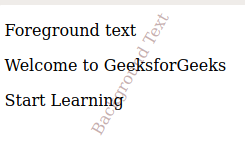# How to use text as background using CSS ?

• Last Updated : 22 Apr, 2020

There are some website designs that require using text as the background. This can be easily achieved with CSS by using the following methods.

• Using absolutely positioned element inside relatively positioned element: The absolutely positioned element inside a relative positioned element with absolute element having lower z-index value gives text appears as the background.

Example:

 ```<``html``>`` ` `<``head``>``    ``<``title``>``        ``Using text as background with CSS``    ```` ` `    ``<``style``>``        ``.container {``            ``position: relative;``        ``}`` ` `        ``.containerbackground {``            ``margin: 3rem;``            ``position: absolute;``            ``top: 0;``            ``left: 0;``            ``bottom: 0;``            ``z-index: -1;``            ``transform: rotate(300deg);``            ``-webkit-transform: rotate(300deg);``            ``color: #c6afaf;``        ``}``    `````` ` `<``body``>``    ``<``div` `class``=``"container"``>``        ``<``div` `class``=``"containerbackground"``>``            ``Background Text``        ````        ``<``p``>Foreground text``        ``<``p``>Welcome to GeeksforGeeks``        ``<``p``>Start Learning``    `````` ` ``

Output:• Using :after pseudo elements: Using :after pseudo elements with :after pseudo elements having lower z-index value make it to appear as background. Use text inside :after pseudo element to make appear text as the background.

Example:

 ```<``html``>`` ` `<``head``>``    ``<``title``>``        ``Using text as background with CSS``    ```` ` `    ``<``style``>``        ``.bgtext {``            ``position: relative;``        ``}`` ` `        ``.bgtext:after {``            ``margin: 3rem;``            ``content: "Background text";``            ``position: absolute;``            ``transform: rotate(300deg);``            ``-webkit-transform: rotate(300deg);``            ``color: rgb(187, 182, 182);``            ``top: 0;``            ``left: 0;``            ``z-index: -1;``        ``}``    `````` ` `<``body``>``    ``<``div` `class``=``"bgtext"``>``        ``<``p``>Foreground text``        ``<``p``>Welcome to GeeksforGeeks``        ``<``p``>Start Learning``    `````` ` ``

Output:• Using :before pseudo elements: Using :before pseudo elements with :before pseudo elements having lower z-index value make it to appear as background. Use text inside :before pseudo element to make appear text as the background.

Example:

 ```<``html``>`` ` `<``head``>``    ``<``title``>``        ``Using text as background with CSS``    ```` ` `    ``<``style``>``        ``.bgtext {``            ``position: relative;``        ``}`` ` `        ``.bgtext:before {``            ``margin: 3rem;``            ``content: "Background text";``            ``position: absolute;``            ``transform: rotate(300deg);``            ``-webkit-transform: rotate(300deg);``            ``color: rgb(187, 182, 182);``            ``top: 0;``            ``left: 0;``            ``z-index: -1;``        ``}``    `````` ` `<``body``>``    ``<``div` `class``=``"bgtext"``>``        ``<``p``>Foreground text``        ``<``p``>Welcome to GeeksforGeeks``        ``<``p``>Start Learning``    `````` ` `       `

Output :My Personal Notes arrow_drop_up